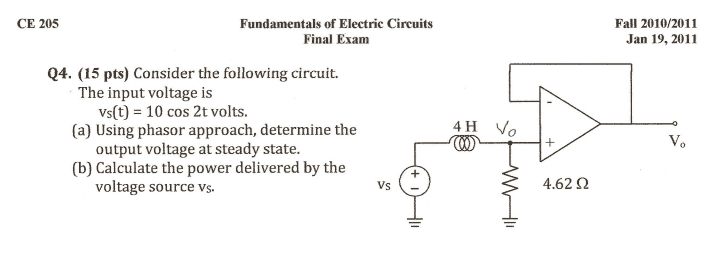# Opamp Circuit Question

## Homework StatementV_- = V_+

## The Attempt at a Solution

There is negative feed back so V- =V+ .... Vout is then equal to the voltage at the positive terminal

So the solution does a voltage divider... finding the voltage at the 4.62 ohm resistor..
However I did KCL at Vo...i1 = i2(i1 being the current going into the positive terminal and i2 being the current flowing down towards the resistor) ====> ((Vo - 10<0)/ j8) = Vo/4.62 ...my final answer for Vo was 5<60 or 2.5 +j4.33..where did I go wrong?

#### Attachments

Last edited by a moderator:

gneill
Mentor
Hi brk51,

Welcome to Physics Forums!

## Homework Statement

View attachment 198279

V_- = V_+

## The Attempt at a Solution

There is negative feed back so V- =V+ .... Vout is then equal to the voltage at the positive terminal

So the solution does a voltage divider... finding the voltage at the 4.62 ohm resistor..
However I did KCL at Vo...i1 = i2(i1 being the current going into the positive terminal and i2 being the current flowing down towards the resistor) ====> ((Vo - 10<0)/ j8) = Vo/4.62 ...my final answer for Vo was 5<60 or 2.5 +j4.33..where did I go wrong?
Your node equation is not correct. On the left side you have the current flowing from the node through the inductor to the source, and on the right you have the current flowing from the node through the resistor. They can't both be flowing out if they are on opposite sides of the equation, since that leaves nothing flowing into the node. You're bound to end up with a sign error.

The way to avoid this sort of slip is to always write all the terms on one side of the equation and set the other side to zero. Choose one direction for the current terms, either all treated as flowing into the node or all flowing out of the node. The math will take care of itself.

So if we take what you've already done and write it as a sum of currents flowing out of the node we have:

##\frac{Vo - 10∠0}{j8} + \frac{Vo}{4.62} = 0##

Gotcha!! So correct answer for Vo should be 5<-60.. then for the second part..finding the power supplied by the Vs --- (10<0 - Vo)/(j8) = I1

Power supplied by source = ((-V * I)/2) cos(thetav - thetai) --- the negative sign because it's supplying (think I might have this wrong..) and divided by two because these are not RMS values...>>>> thetav = 0 theta i = -60..thus cos(60) >>> so ((10)*(1.082))/2) * cos(60) = 2.7 W or -2.7 W ??

Last edited:
Homework Helper
Gold Member
2020 Award
Did you get that ## \omega=2 ## for this problem and ## L=4 ## so that ## Z_L=j \omega L=j(2)(4) ##? ## V_s=10 ##... Homework helpers are not allowed to give the answer. Please show your work and it would be easier to comment on.

Did you get that ## \omega=2 ## for this problem and ## L=4 ## so that ## Z_L=j \omega L=j(2)(4) ##? ## V_s=10 ##... Homework helpers are not allowed to give the answer. Please show your work and it would be easier to comment on.
yes.. and see my post above

Homework Helper
Gold Member
2020 Award
I would just write ## V_R=V_s (R/(R+j \omega L)) ##. This one is very straightforward. It's an op-amp buffer circuit and no current flows through(i.e. across the infinite input impedance of) the ideal op-amp.

I would just write ## V_R=V_s (R/(R+j \omega L)) ##. This one is very straightforward. It's an op-amp buffer circuit and no current flows through the ideal op-amp.
Thats what the solution did, easy v-divider..but I just went with my first instinct...

However, my question is now on part 2>>> I got Psupplied = (10)*(1.082))/2) * cos(60) = 2.7 W

•Homework Helper
Gold Member
2020 Award
The voltage across the resistor is in phase with the current. There is no power loss in the inductor. You just need to compute ## P=V_R^2/(2R) ##. (The factor 2 in the denominator to make it r.m.s. of ## V_R^2 ##.) You could also take the r.m.s. voltage out of the source multiplied by the r.m.s. current and multiplied by the cosine of the phase angle, but the first method is quicker. They should give the same result in both cases. ## \\ ## I think my arithmetic is correct, and it agrees with your answer that ## P=2.7 ## W.

Last edited:
Gracias... last question.. if it is asking for power supplied..does that mean the 2.7 W is negative? How do you know when to make it negative?

•